# The Probability of Bankruptcy

This entry is part 3 of 4 in the series Risk of Bankruptcy

In the simulation we have for every year calculated all four metrics, and over the 250 runs their mean and standard deviation. All metrics is thus based on the same data set. During the forecast period the company invested heavily, financed partly by equity and partly by loans. The operations admittedly give a low but fairly stable return to assets. It was however never at any time in need for capital infusion to avoid insolvency. Since we now “know” the future we can judge the metrics ability to predict bankruptcy.

A good metric should have a low probability of rejecting a true hypothesis of bankruptcy (false positive) and a high probability of rejecting a false hypothesis of bankruptcy (false negative).

In the figures below the more or less horizontal curve gives the most likely value of the metric, while the vertical red lines indicate the 90% event space. By visual inspection of the area covered by the red lines we can get an indication of the false negative and false positive rate.

The Z-Index shows an increase over time in the probability of insolvency, but the probability is very low for all years in the forecast period. The most striking effect is the increase in variance as we move towards the end of the simulated period. This is caused by the fact that uncertainty is “accumulated” over the forecast period. However, according to the Z-index, this company will not be endangered inside the 15 year horizon.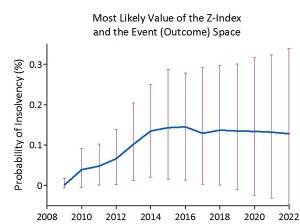In our case the Z-Index correctly identifies the probability of insolvency as small. By inspecting the yearly outcomes represented by the vertical lines we also find an almost zero false negative rate.

The Z-score metrics tells a different story. The Z’’-score starts in the grey area and eventually ends up in the distress zone. The two others put the company in the distress zone for the whole forecast period.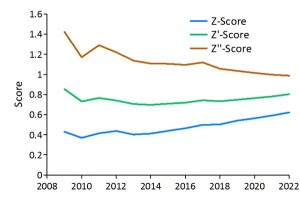Since the distress zone for the Z-score is below 1.8, a visual inspection of the area covered by the red lines indicates that most of the outcomes fall in the distress zone. The Z-score metrics in this case performs type II errors by giving false negative judgements. However it is not clear what this means – only that the company in some respect is similar to companies gone bankrupt.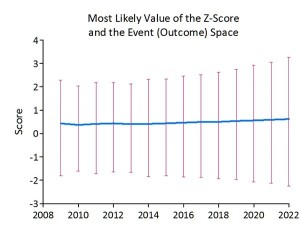If we look at the Z metrics for the individual years we find that the Z-score have values from minus two to plus three, in fact it has a coefficient of variation ranging from 300% to 500%. In addition there is very little evidence of the expected cumulative effect.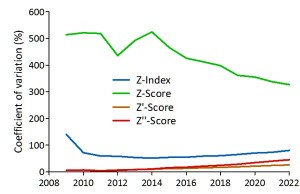The other two metrics (Z’ and Z’’) shows much less variation and the expected cumulative effect.  The Z’-score outcomes fall entirely in the distress zone, giving a 100% false negative rate.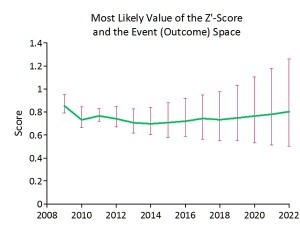The Z’’-score outcome falls mostly in the distress zone below 1.1, but more and more falls in the grey area as we move forward in time. If we combine the safe zone with the grey we get a much lower false negative rate than for both the Z and the Z’ score.It is difficult to draw conclusions from this exercise, but it points to the possibility of high false negative rates for the Z metrics. Use of ratios in assessing a company’s performance is often questionable and a linear metric based on a few such ratios will obviously have limitations. The fact that the original sample consisted of the same number of healthy and bankrupt companies might also have contributed to a bias in the discriminant coefficients. In real life the failure rate is much lower than 50%!

Series Navigation<< Predicting BankruptcyCredit Risk >>